# Understand the statistics behind A/B testing — an intuitive way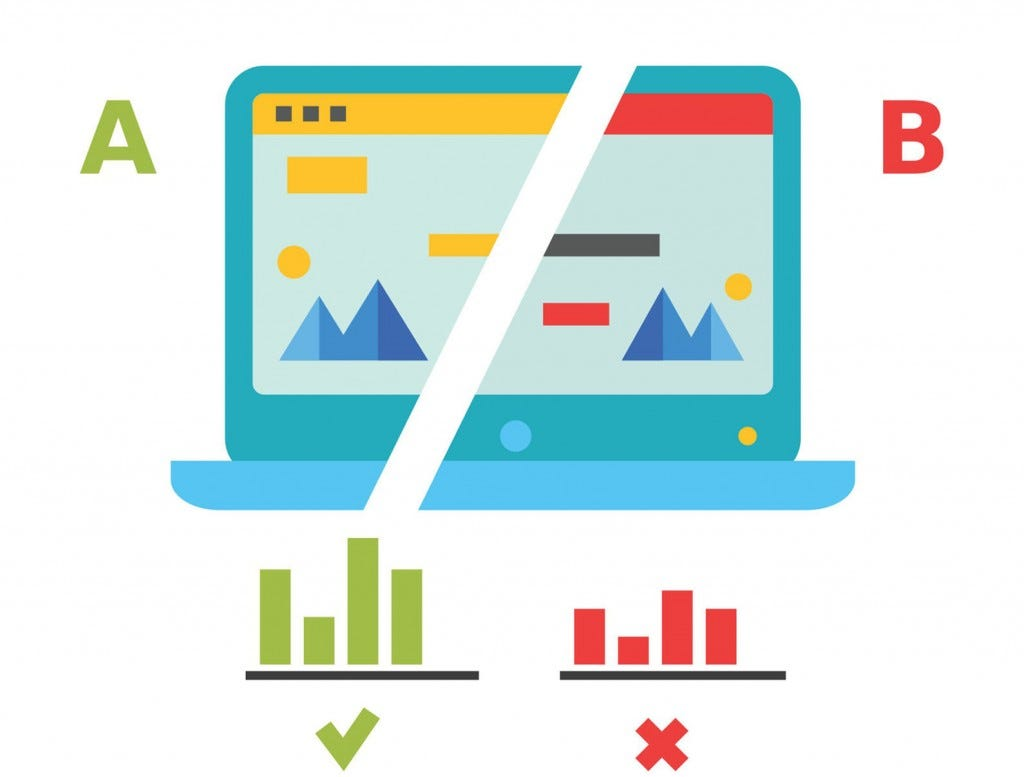Image Source: Invesp

The goal of A/B testing is to gather data to support decision making. While looking at the analytics dashboards and metrics, the most common question a data scientist, product manager or consultant encountered is: how could I translate the A/B testing results into a launch/no-launch decision? To properly answer this question, we need to take into consideration of both the conclusion drawn from the measurement data and the broader context, such as trades offs between metrics, various costs and risks.

In this article, we will only be focusing on the statistics behind designing an A/B test and interpreting the results. As the purpose of this post is to explain the fundamentals of statistical testing in a more intuitive way, I will only include the math if it’s necessary. (we don’t want to get overwhelmed by a whole page of equations and fail to grasp the ideas behind the basic concepts) But I do encourage you to check out the mathematical fundamentals behind it if you want to dive deeper into a certain concept.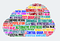cerdited: Author

Find these words in the picture above daunting yet familiar? These are the concepts you will be able to connect together by solving this question with me:

# What you will learn:

1. Design an experiment and formulate the hypotheses
2. Simulate an A/B test and evaluate the distributions of the sample data
3. Obtain test statistics, p-value and confidence interval
4. Statistical power and type I & II error

# 1. Null Hypothesis and Alternative Hypothesis

Imagine we are running an A/B test for a retail company to evaluate if a change in its sign-up bottom on its website will lead to increase in account signups from users. Before we start running the experiment, we will need to define the baseline conversion rate and the desired lift (differences between groups). In our case, let’s assume that we currently have 20 out of 100 users who visited our website signed up an account, and we want to use our test to confirm that the new change we made in our sign-up bottom will result in at least a 2% increase in the sign-up rate.

The number of users participating in an A/B test (sample) makes up a small percentage of the total user base (population). Users are randomly selected and assigned to either a control group or a treatment group. The sample size of each group depends on the power of the test and the effect size that we will learn later on in this article. Here, we will assign 1000 users for each group initially and serve the current webpage to the control group and the webpage with new signup bottom to the test group.

We are running a hypothesis test here: A hypothesis test evaluates two mutually exclusive statements about a population to determine which statement is best supported by the sample data. These two statements are called the null hypothesis and the alternative hypothesis.

In every experiment, there is an effect or a difference (d) between groups that the researchers are testing, and in our case: the benefits of a different sign-up bottom in our website. Typically, the null hypothesis Ho states that the true effect size equals to zero — that there is no difference between groups. The alternative hypothesis Ha states that the true effect size does not equal to the null hypothesis value — which could be in one direction (greater or less than zero) or both directions (not equal to zero) depending on the type of tests we are conducting.

# 2. Generate sample data and plot their distributions

Here we will generate random numbers to simulate real online experiment log data. The converted column indicates the number of users signed up for an account. The group column represents the control group and the treatment group with A and B, respectively. The python scripts for generating and summarizing the data can be found at my GitHub repo here.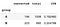Overview of sample data

The difference in conversion rate between group A and B is 0.033 (0.2247–0.1924), which is slightly higher than the 0.02 difference we initially wanted to observe. So here comes the question: Is this 0.033 difference we measured give us enough evidence to roll out the new sign-up bottom? And if so, how confident are we in our judgement?

To answer this question, let’s first look at the distributions of the control & treatment groups. We can assume that the distributions of both groups are binomial since our experiment is a series of Bernoulli trials — a random experiment that has only two outcomes (usually called a “Success” or a “Failure”)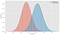binomial distribution of control & treatment group

We can tell from the graphic that the treatment group converted more users than the control group, but if we look at the peak of each distribution, the control group has a higher probability to convert at some levels. To obtain a more direct comparison, we should focus on the conversion rate instead of the converted numbers.

## Bernoulli Distribution

First, recall our experiments in two groups are two Bernoulli trails, and their outcomes follow a Bernoulli distribution. The two possible outcomes in Bernoulli distributions are labeled by n=1 (success, in our case: converted) and n=0 (failure), in which n=1 occurs with probability p (in our case: conversion rate) and n=0 occurs with probability 1-p. The probability mass function (PMF) of a Bernoulli distribution is defined as:

There are two properties of a Bernoulli distribution: mean and variance

Remember, our task here is to approximate μ (the population mean; in our case, the conversion rate of all users) based on ( the sample mean; in our case, the conversion rate of the experiment groups). According to the Central Limit Theorem(CLT):

• The distribution of sample means (also can be represented by) approximates a normal distribution as the sample size gets larger;
• The sample means will be approximately equal to the mean of the population μ.

Applying the above steps, we have two normal distribution for the mean conversion rate of control and treatment groups: p_A and p_B .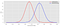Normal distributions of control & treatment group for conversion rate

The dashed lines represent the mean conversion rate for each group, and the distance between the two lines d represents the mean of the difference between the control and treatment group. denotes the distribution of the difference.

In probability theory, the sum of the normally distributed independent random variables is also normally distributed. Thus, follows normal distribution.

Okay, enough math 🚫. Why do we want to calculate and know its distribution? Recall the hypotheses we came up with at step1:

We want to find enough evidence to reject the null hypothesis, this means that the mean of the alternative hypothesis will be located at a different value than the mean followed by the null hypothesis (which is 0 in our case). It will make more sense if we plot the hypotheses out.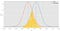Distributions of the null & alternative hypothesis

We can tell that the mean difference between treatment and control group (shown as the two vertical dash lines in the graph) seems higher under the alternative hypothesis than the null hypothesis. But what if the difference falls into the shaded area? Can we still determine which distribution it came from?

Well, the truth is that after running our experiment, we get a resulting conversion rate for both groups, and we end up with one result — the difference between the conversion rates. However, we can never be 100% sure about which population the difference is deriving from — the null or the alternative hypothesis.

What if I tell you there is a way of rejecting the Null hypothesis and conclude that our experiment has an effect — if the probability of observing such difference is relatively small assuming there is no difference. Wait, so what we need to do is to find that “probability” and determine if it’s small enough, right?

# 3. Test statistics, p-value and confidence interval

P-value by definition: P-values are the probability that a sample will have an effect at least as extreme as the effect observed in your sample if the null hypothesis is correct. To reject our null hypothesis, we hope that the p-value is small enough.

How small is small enough?

The scientific standard is to use a p-value less than 0.05, 0.05 here is the significance level(α), meaning that if there is truly no effect, we can correctly infer there is no effect 95 out of 100 time — the result is statistically significance. The equivalent way of accessing statistical significance is to find the confidence interval (CI).

A 95% CI is the range that covers the true difference 95% of the time, and 95% here is the confidence level. In hypothesis testing, A 95% confidence level corresponds to a significance level of 0.05.Relationship between Significance Level and Confidence Level

The CI for our null hypothesis is shown as below.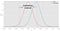Confidence Interval for null hypothesis

Let’s pick 0.05 as our significance level(α), our next step is to decide if we should reject the null hypothesis base on our significance level and sample data. There are typically two ways to do that:

• Compute test statistic: if test statistic > critical value, then reject the null hypothesis
• Compute p-value: if p-value < α, then reject the null hypothesis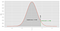Two-sample Z test (one-sided)

We performed the Two-sample Z test (will cover it in other post, just take it as a way of testing a hypothesis for now), we obtained the critical value: z=1.645, which is smaller than the z test statistic: 1.780. Thus we can reject the null hypothesis.

If we choose to compute the p-value, we will reach the same conclusion: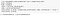z statistic & p-value for two-sample Z test(one-sided)

Our p-value in this case is 0.0376, less than the 0.05 significance level. Thus, we can reject the null hypothesis and conclude that the new webpage design increases the conversion rate by at least 1%.

# 4. Type I error, Type II error, statistical power

When we set the significance level to 0.05, it indicates that we are willing to accept a 5% chance that we would be wrong when we reject the null hypothesis that is in fact true (type I error). The probability of making a type I error is α = 0.05.

What if we fail to reject a null hypothesis that is false when our p-value is larger than 0.05? That is called a type II error. The probability of making a type II error is referred to as beta, and it’s the area segmented by the significance level under the alternative hypothesis.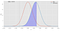Type II error

If the purple shaded area is the probability of us failing to reject the null hypothesis when it is false, then the right side under the curve would be the probability of rejecting the null hypothesis when it is false — that is called: the power of a test (statistical power) as shown in the green area below: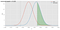Statistical power of the test

Researchers generally choose 0.8 as a standard of adequacy for statistical power. You might think our statistical power is quite insufficient in comparison — but remember: we are conducting the experiment on a very small set of samples, and A/B tests usually are deployed to far more number of users in real life.

If we simply increase the users in each group from 1000 to 2000, we can already observe a leap in our statistical power as shown below.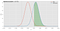Statistical power with increased sample number

# End words

Now you understand how to leverage p-value to evaluate the results from an A/B test, and hope you enjoy it so far. An end-to-end A/B test includes designing, deploying, and analyzing an experiment. This article only walked you through the analysis part from a statistical point of view. For question such as: How can we obtain the sample size that make sure the statistical power of our test is adequate? How should we randomize the samples? How long should we run an experiment? I will cover these in my next post.

The best way to learn is through recall and practice. Try explain this to yourself : what is p-value? When should I reject a null hypothesis, and why? 🥑 Leave me a common below if you have any question, and if you find this article helpful please give me a 👏.

# References:

Data Science, Cloud Computing, ML, Quantum Computing | Fitness enthusiast | Avocado Lover 🥑

## More from Eva He

Data Science, Cloud Computing, ML, Quantum Computing | Fitness enthusiast | Avocado Lover 🥑